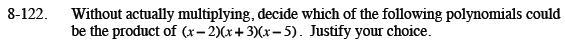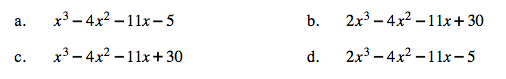Home > CCA2 > Chapter 8 > Lesson 8.3.1 > Problem8-122

8-122.
1. Without actually multiplying, decide which of the following polynomials could be the product of (x − 2)(x + 3)(x − 5). Justify your choice. Homework Help ✎

1. x3 − 4x2 − 11x − 5

2. 2x3 − 4x2 − 11x + 30

3. x3 − 4x2 − 11x + 30

4. 2x3 − 4x2 − 11x − 5If you expanded the original polynomial, what would be the first term? What would be the last term?